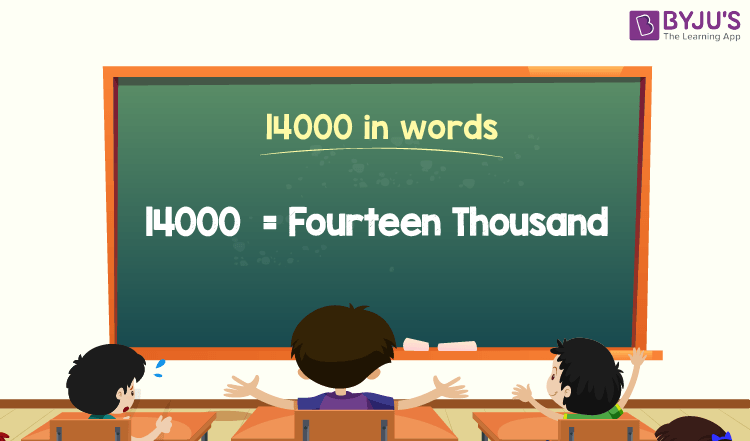# 14000 in Words

14000 in words is Fourteen Thousand. The number name of 14000 can be written using the ones, tens, hundreds, thousands place of a number. Thus, the place value chart helps to write the number 14000 in words.

 14000 in Words: Fourteen Thousand

In this article, we will learn how to write the number 14000 in words, and look at the solved examples in detail.

## How to Write 14000 in Words?The number 14000 in words is written using the place value of a number. First, identify the place value of each digit in the given number using the place value chart. Here, the number is 14000. Hence, identify the place value of each digit of 14000 to write the number’s name.

For a number 14000,

In one’s place, the digit = 0

In ten’s place, the digit = 0

In hundred’s place, the digit = 0

In thousand’s place, the digit = 14

Thus, the place value chart for 14000 is:

 Thousands Hundreds Tens Ones 14 0 0 0

Therefore, the number 14000 in words is Fourteen Thousand.

### Examples

Example 1:

Find the value of 11000 + 3000. Describe the value in words.

Solution:

Given expression: 11000 + 3000

⇒ 11000 + 3000 = 14000

So, the value of 11000 + 3000 is 14000.

Hence, 14000 in words is fourteen thousand in words.

Example 2:

Express the value of twenty thousand minus six thousand in words.

Solution:

Twenty thousand = 20000

Six thousand = 6000

Twenty thousand minus six thousand = 20000 – 6000= 14000

Hence, the value of twenty thousand minus six thousand is fourteen thousand in words.

## Frequently Asked Questions on 14000 in Words

Q1

### Write 14000 in words.

14000 in words is fourteen thousand.

Q2

### Express the value 21000 – 7000 in words.

Simplifying 21000 – 7000, we get 14000. Hence, 14000 in words is fourteen thousand.

Q3

### How to write fourteen thousand in numbers?

Fourteen thousand in numbers is 14000.